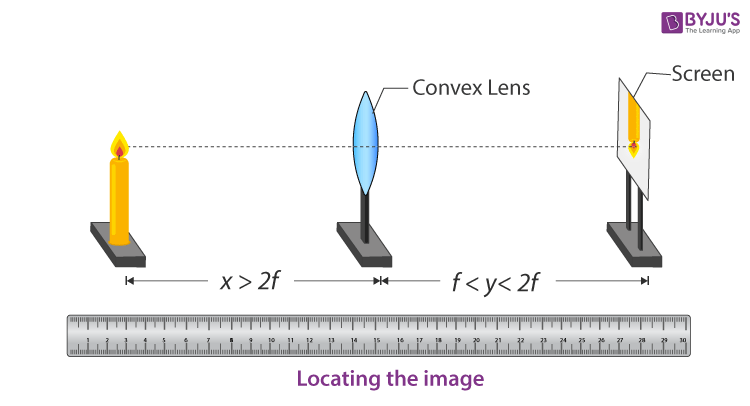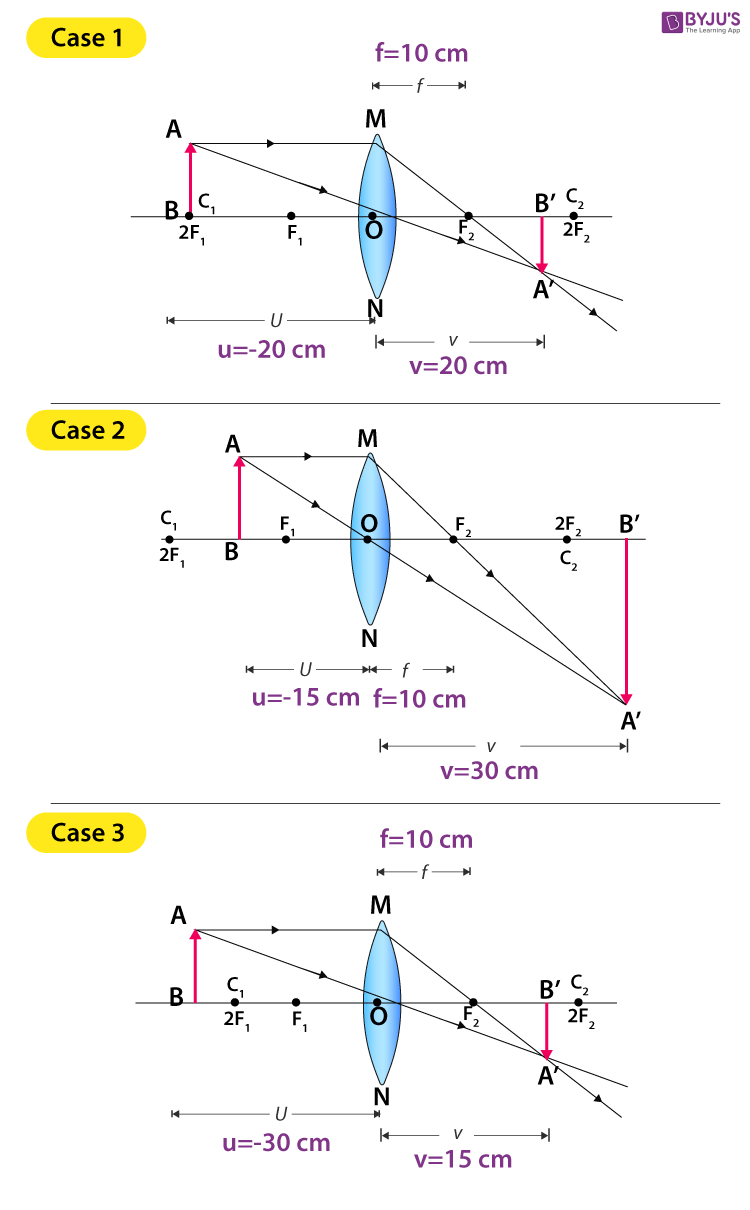Jet Set Go! All about Aeroplanes Jet Set Go! All about Aeroplanes

# To Find The Image Distance For Varying Object Distances In Case Of A Convex Lens With Ray Diagrams

The lens is a transparent material which is bound by two surfaces. It has a principal axis, principal focus, centre of curvature of lens, aperture and optical centre.There are two types of lenses, they are a convex lens and a concave lens. The images obtained from these lenses can be either a real image or a virtual image. Below is an experiment to find the image distance for varying object distances of a convex lens with ray diagrams.

## Aim

To find the image distance for varying object distances in case of a convex lens and drawing corresponding ray diagrams to show the nature of the image formed.

## Theory

### What is a convex lens?

A convex lens is defined as a lens which is thick at the centre and thin at the edges. A convex lens is also known as a converging lens as it converges the light beam incident on it. There are three types of convex lens:

• Double convex lens
• Plano-convex lens
• Concavo-convex lens

### What is lens formula?

Lens formula is defined as the relationship between object distance (u), image-distance (v) and the focal length (f). Following is the mathematical representation of lens formula:

 $$\begin{array}{l}\frac{1}{f}=\frac{1}{v}-\frac{1}{u}\end{array}$$

Where,

• f is the focal length of the lens
• v is the distance of the image from the optical centre of the lens
• u is the distance of an object from the optical centre of the lens

## Materials Required

Following are the materials required for the experiment:

1. A convex lens with a focal length of 12-20 cm.
2. Measuring scale
3. Optical bench
4. A needle
5. A candle

## Experimental setup## Procedure

1. Place the convex lens on a lens holder, placing the screen on the other side of the lens.
2. Try focusing the image to get a sharp, clear and inverted image on the screen. The focal length that is obtained is a rough focal length which can be measured using a meter scale.
3. The position at which the lens is fixed, mark it as O.
4. Once the focal length is calculated, mark point F on both the sides of the lens.
5. Mark a point 2F, as the distance between the lens is double the focal length of the lens.
6. Place the candle at a distance beyond 2F and adjust the height of the centre of the lens with the height of the flame.
7. To obtain a sharp image of the candle flame in the convex lens, adjust the position of the screen and record the observations.
8. Record the observations by placing the needle or lighted candle at 2F.
9. Record the observations by shifting the object between F and 2F.
10. Record the observations by placing the object at F.
11. Record the observations by placing the object between O and F.
12. Draw the ray diagrams for all the positions of the object.

## Ray Diagrams## Observation Table

 Sl. no Postion of the optical centre O of the lens l (cm) Position of candle a (cm) Position of screen s (cm) Distance between lens and candle (object distance) u=a-l (cm) Distance between lens and screen (image distance) v=s-l (cm) Focal length (l) 1 50 30 70 -20 20 10 cm 2 50 35 80 -15 30 10 cm 3 50 20 65 -30 15 10 cm 4 50 40 No image obtained on screen -10 Infinity 5 50 45 No image obtained on screen -5 Virtual image obtained and cannot be taken on screen

## Calculations

Following is the calculations of f for different cases explaining the focal length of the given lens is 10cm:

 Case 1: $$\begin{array}{l}\frac{1}{f}=\frac{1}{v}-\frac{1}{u}\Rightarrow \frac{1}{f}=\frac{1}{20}-(\frac{1}{-20})=\frac{2}{20}=\frac{1}{10}\Rightarrow f=10\;cm\end{array}$$ Case 2: $$\begin{array}{l}\frac{1}{f}=\frac{1}{v}-\frac{1}{u}\Rightarrow \frac{1}{f}=\frac{1}{30}-(\frac{1}{-15})=\frac{3}{30}=\frac{1}{10}\Rightarrow f=10\;cm\end{array}$$ Case 3: $$\begin{array}{l}\frac{1}{f}=\frac{1}{v}-\frac{1}{u}\Rightarrow \frac{1}{f}=\frac{1}{15}-(\frac{1}{-30})=\frac{3}{30}=\frac{1}{10}\Rightarrow f=10\;cm\end{array}$$

## Result

 S.no Position of the object Position of the image Relative size of the image Nature of the image 1 At 2F1 At 2F2 Same size Real and inverted 2 Between F1 and 2F1 Beyond 2F2 Enlarged Real and inverted 3 Beyond 2F1 Between F2 and 2F2 Diminished Real and inverted 4 At focus F1 At infinity Infinitely large or highly enlarged Real and inverted 5 Between focus F1 and optical center O On the same side of the lens as the object Enlarged Virtual and erect 6 At infinity At focus F2 Highly diminished, point-sized Real and inverted

The focal length of the given lens is 10cm.

## Precautions

• The convex lens must have the focal length between 15 to 20 cm.
• The convex lens must have a small aperture.
• To avoid the flickering of the candle flame, the experiment can be conducted in calm air.
• Perform the experiment in a dark room so as to obtain a distinct and sharp image of the candle flame.
• Image screen shouldn’t be shaky.

## Viva Questions

Q1. What is a lens?
Ans: Lens is defined as a transparent material with curved sides on both the sides or one curved and one plane surface.

Q2. Define power of a lens.
Ans: Power of a lens is defined as the reciprocal of the focal length. The mathematical formula is given as:

 $$\begin{array}{l}P=\frac{1}{f}\end{array}$$

Where,

• P is the power of a lens
• f is the focal length

Q3. What is one dioptre?
Ans: One dioptre is defined as a unit of measurement of the optical power of a curved mirror or a lens. It is given as follows:

 1 dioptre = 1m-1

Q4. What is meant by principal of axis?
Ans: Principle of axis is defined as the line passing through the centre of the lens or a spherical mirror.

Q5. What is the focal length of a lens?
Ans: Focal length of a lens is defined as the distance between the principal focus and the optical centre.